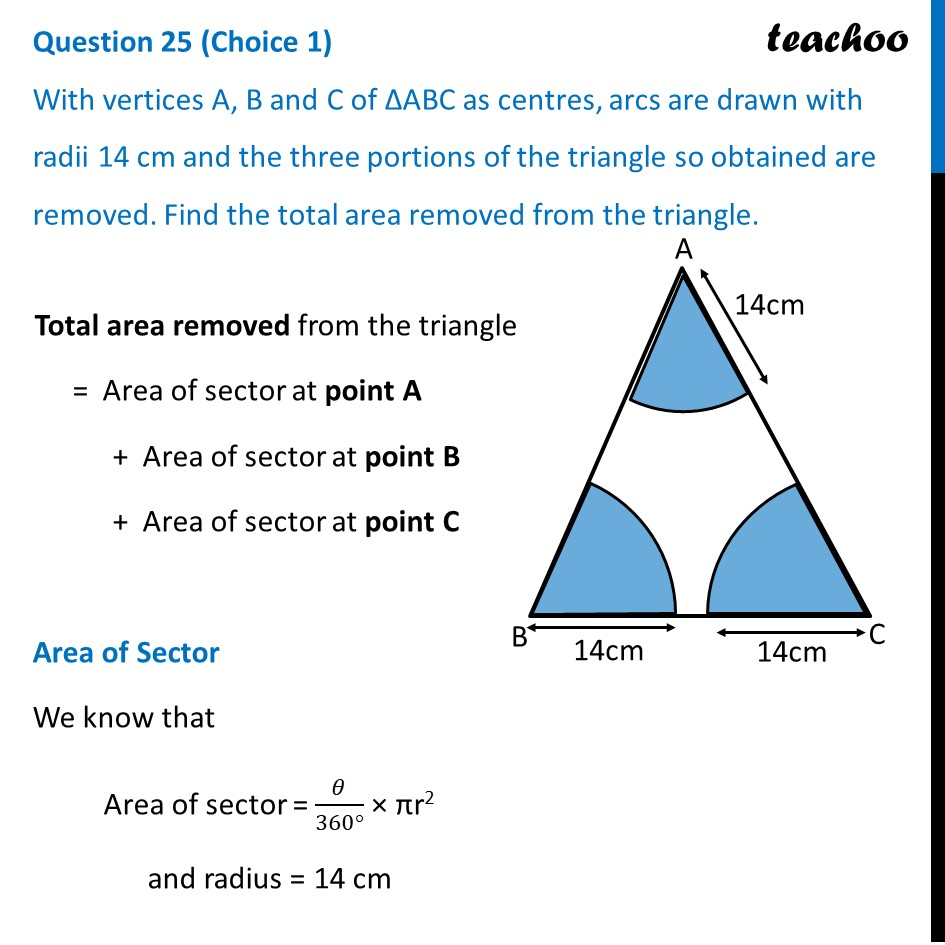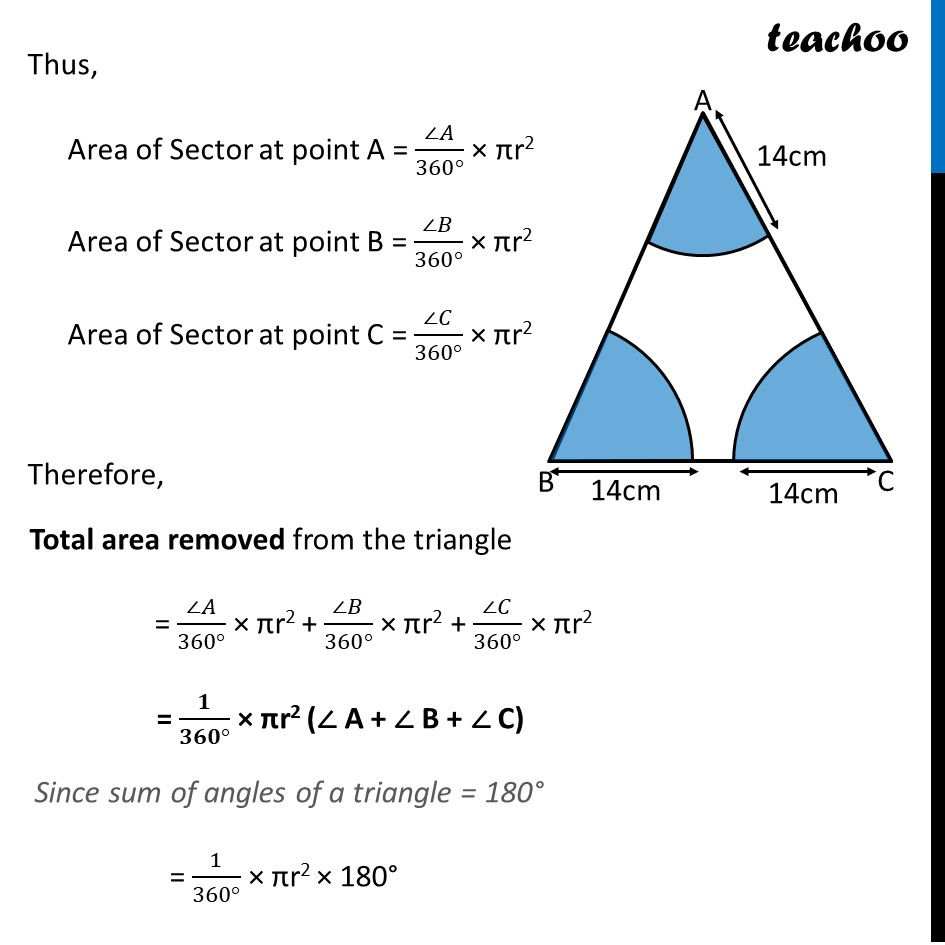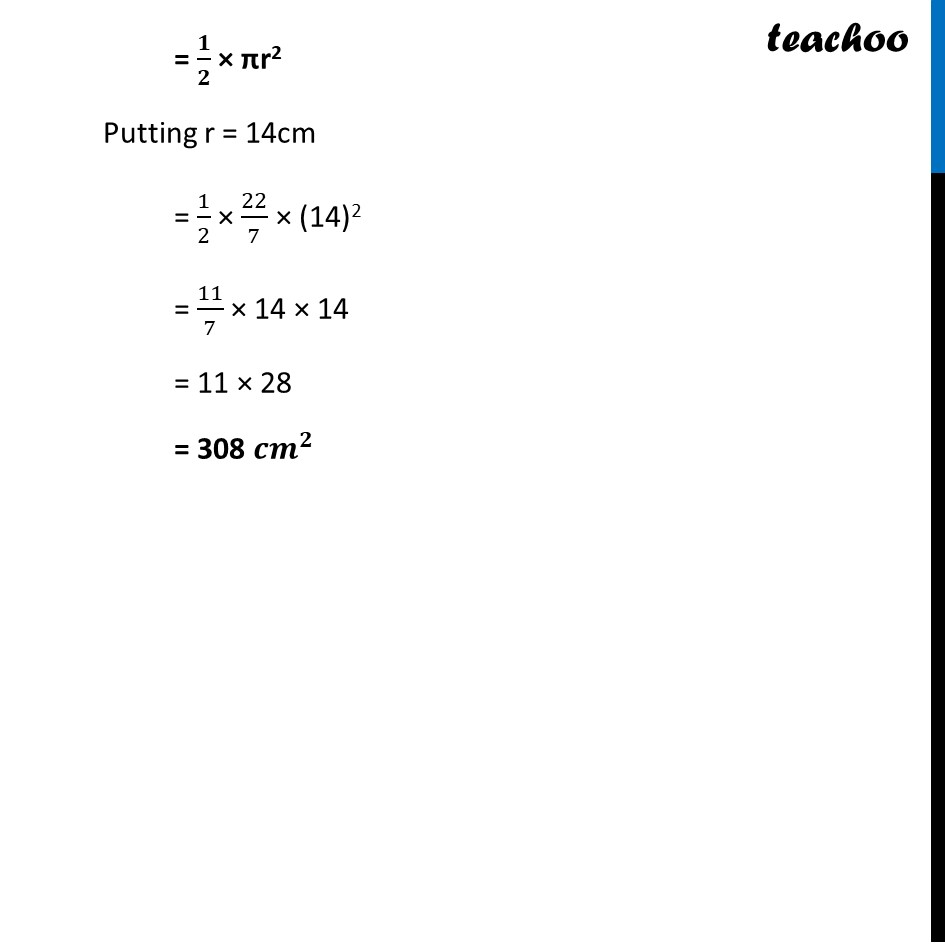CBSE Class 10 Sample Paper for 2024 Boards - Maths Standard

Class 10
Solutions of Sample Papers for Class 10 Boards

## With vertices A, B and C of ΔABC as centres, arcs are drawn with radii 14 cm and the three portions of the triangle so obtained are removed. Find the total area removed from the triangle.Learn in your speed, with individual attention - Teachoo Maths 1-on-1 Class

### Transcript

Total area removed from the triangle = Area of sector at point A + Area of sector at point B + Area of sector at point C Area of Sector We know that Area of sector = 𝜃/(360°) × πr2 and radius = 14 cm Thus, Area of Sector at point A = (∠𝐴)/(360°) × πr2 Area of Sector at point B = (∠𝐵)/(360°) × πr2 Area of Sector at point C = (∠𝐶)/(360°) × πr2 Therefore, Total area removed from the triangle = (∠𝐴)/(360°) × πr2 + (∠𝐵)/(360°) × πr2 + (∠𝐶)/(360°) × πr2 = 𝟏/(𝟑𝟔𝟎°) × πr2 (∠ A + ∠ B + ∠ C) Since sum of angles of a triangle = 180° = 1/(360°) × πr2 × 180° = 𝟏/𝟐 × πr2 Putting r = 14cm = 1/2 × 22/7 × (14)2 = 11/7 × 14 × 14 = 11 × 28 = 308 〖𝒄𝒎〗^𝟐